# Impact of the Integration of a STATCOM Controlled by LQG/H2 Regulator in an Energy System

Impact of the Integration of a STATCOM Controlled by LQG/H2 Regulator in an Energy System

Mohammed MekkiHouari Merabet Boulouiha Ahmed Allali Mouloud Denai

Department of Electrical Engineering, LDDEE Laboratory, University USTO-MB, Oran 31000, Algeria

Department of Electrical Engineering, SCAMRE Laboratory ENPO-MA, Oran 31000, Algeria

School of Engineering & Computer Science, University of Hertfordshire, Hertfordshire AL10 9AB, UK

Corresponding Author Email:
mekki.doct@gmail.com
Page:
361-370
|
DOI:
https://doi.org/10.18280/ejee.230502
8 July 2021
|
Accepted:
26 September 2021
|
Published:
31 October 2021
| Citation

OPEN ACCESS

Abstract:

Nowadays, the reactive power consumption is becoming a serious problem for electricity network management. To overcome this problem, several solutions are proposed in the literature. In the present study, the static reactive power compensator (STATCOM) solution is used to keep the network voltage within its rated range. The STATCOM is modeled in the axes of Park reference frame and is driven controlled by a SVPWM strategy. Its control scheme is based on a multivariable Linear Quadratic Gaussian (LQG/H2) controller, which has the advantage of being applied to systems whose condition is not measured. Simulations are performed using the MATLAB/SIMULINK software. Results are presented, compared and discussed.

Keywords:

LQG/H2 control, NPC inverter, power quality, reactive power, SVPWM, STATCOM, Three-level, THD

1. Introduction

In recent years, electric power quality has become a primary concern for both network operators and consumers due to the large-scale deployment of renewable energy technologies and increased utilization of power electronics and nonlinear loads. Power quality is assessed based on a number of criteria such as sinusoidal voltage waveform with rated amplitude and frequency.

Changes in voltage amplitude and degradation of the power factor are the consequences of reactive energy consumption. The increased use of this energy causes transmission line disruptions. The voltage at each point in an ideal AC system must be constant and free of harmonics, with a power factor close to unity. These criteria, on the other hand, must be independent of the characteristics of the user load. Only if the loads are equipped with a reactive energy compensation system can this be assured. and it is imperative that this compensating device responds quickly to changing reactive power demands to keep the voltage within acceptable ranges throughout the distribution network. Various reactive power compensation technologies have been in operation over the past years especially that thyristors-based devices such as the TCR (Thyristor Controlled Reactor), TSC (Thyristor Switched Capacitor) and SVC (Static Var Compensator).

The TCR is composed of an inductor connected with two thyristors connected in anti-parallel and each thyristor is controlled during a half period. Modifying the firing angle of thyristors results in a variation of the reactance control as a function of the load demand [1-3]. Because of the inductor, the current flowing through the TCR is strongly inductive. TSCs are in general, composed of dimmers each controlling a bank of capacitors. Because of the capacitor, the current will always be leading the voltage and therefore imposing a reactive power flow to the network .

The combination of TCR and TSC systems yields to SVC hybrid compensator. By adjusting the conduction period of the thyristors, the circuit becomes similar to variable source of reactive power [5-9]. Whereas, these devices are considered as a major source of undesirable harmonics .

Taking profit of progress in the power electronics field, self-commutated devices such as IGBT, MOSFET, GTO, etc. have been adopted for the design of voltage source inverter (VSC)-based static var compensator devices such as the STATCOM (Static synchronous compensator). The STATCOM is operated as a shunt-connected SVC and provides a flexible response to changing loads by injecting or absorbing reactive power instantly to improve the stability and performance of the grid.

To reduce the rate of harmonic distortion of the inverter output voltage, several multilevel inverter topologies have been proposed in the literature [10-17].

According to Mohamed et al. , the performance of a grid-connected wind farm with and without a STATCOM was studied. The results have shown an improvement in the power quality of the wind farm with the STATCOM. The main effect of STATCOM has been observed when a three-phase fault applies to the network. Yuvaraj et al. , Liu and Wang , discussed the use of STATCOM to improve the quality of a wind energy system connected to the grid. In works Chavhan et al.  and Ilango et al. , an Icosφ algorithm is applied to the STATCOM for reactive power compensation and network power factor enhancement. A control technology for the STATCOM has been proposed by Sundaram et al. , for commercial wind turbines to increase the device's power capability and improve its power quality.

Ahsan and Siddiqui  presented a MATLAB/Simulink model of a 12 MW wind farm associated with a STATCOM. Zhang and Li  established a simplified algorithm of PWM model estimation for STATCOM based by cascade converter.

The results show that the STATCOM was able to improve the power quality of the wind farm connected to the AC transmission system.

In this paper, a STATCOM based on a three-level NPC (Neutral Point Clamped) inverter, controlled by a Space Vector Pulse Width Modulation (SVPWM) technique, is employed for reactive power compensation of a grid-connected industrial load. The proposed control scheme is based on a multi-variable Linear Quadratic Gaussian (LQG / H2) regulator and is used to simultaneously control the DC side voltage and reactive power flow.

The rest of the article is structured as follows: The modeling and STATCOM circuit based on a three-level NPC inverter connected to the three-phase network are presented in Section 2. The SVPWM technique is presented in Section 3. A multivariable control synthesis based on LQG / H2 has been introduced in the same section. The simulation results and discussions are presented in Section 4. Section 5 summarizes the conclusions of this contribution and the objectives achieved.

2. Modeling of the STATCOM

Figure 1 illustrates the proposed circuit of STATCOM compensator, by using the technique of SVPWM for DC-AC conversion. Different blocks constituting the configuration of the multi-variable control circuit of STATCOM are shown. Measurements of the compensator currents in the d and q axes of Park and the voltage on the DC side will be used to calculate the control laws. DC voltage changes as well as reactive energy are possible with the multi-variable regulator, where the output of this regulator provides two outputs. The phase α is the first output, which is added to the network's pulsation ωt. The second output is the inverter's conversion ratio between the fundamental of the output voltage and the input DC voltage (D). With the latter, the modulation index (MI) can be calculated using the following formula:

$M I=\sqrt{\frac{2}{3}} D$    (1)

The following equation gives the pulse functions:

$S=\left[\begin{array}{c}S_{a} \\ S_{b} \\ S_{c}\end{array}\right]=M I\left[\begin{array}{c}\sin (\omega t+\alpha) \\ \sin \left(\omega t-\frac{2 \pi}{3}+\alpha\right) \\ \sin \left(\omega t+\frac{2 \pi}{3}+\alpha\right)\end{array}\right]$    (2)

The mathematical model of current compensator STATCOM in Park axes (d-q) is:

$\left[\begin{array}{cc}L_{S} p+R_{S} & \omega L_{S} \\ -\omega L_{S} & L_{S} p+R_{S}\end{array}\right]\left[\begin{array}{c}i_{c q} \\ i_{c d}\end{array}\right]=\left[\begin{array}{c}-V_{S} \sin \alpha \\ V_{S} \cos \alpha-D v_{d c}\end{array}\right]$    (3)

where, VS is the source's rms line voltage, D is the inverter conversion ratio between the fundamental of the output voltage and the DC input voltage, α is the phase angle between the network voltages and the inverter's output voltages, p is Laplace operator.

The continuous side equation in (q-d) axes is written as follows:

$\frac{d v_{d c}}{d t}=\frac{D}{C_{S}} i_{c d}$    (4)

The final multivariate model of STATCOM is given by:

$\left\{\begin{array}{l}x(t+1)=A x(t)+B u(t) \\ y=C x(t)\end{array}\right.$    (5)

With:

$A=\left(\begin{array}{ccc}-\frac{R_{s}}{L_{s}} & -\omega & 0 \\ \omega & -\frac{R_{s}}{L_{s}} & -\frac{D}{L_{s}} \\ 0 & \frac{D}{C_{s}} & 0\end{array}\right), \quad B=-\frac{1}{L_{S}}\left(\begin{array}{cc}-V_{S} & 0 \\ 0 & v_{d c 0} \\ 0 & 0\end{array}\right)$

$C=\left(\begin{array}{ccc}-1 & 0 & 0 \\ 0 & 0 & 1\end{array}\right), x=\left[\begin{array}{lll}i_{c q} & i_{c d} & v_{d c}\end{array}\right]^{T}, u=\left[\begin{array}{ll}\alpha & D\end{array}\right]^{T}$

The detail of the modeling is mentioned in APPENDIX A.

where A denotes the state matrix, B denotes the input matrix, C denotes the output matrix, and x, u, and y denote the state vector, input vector, and output vector, respectively.

## 1.png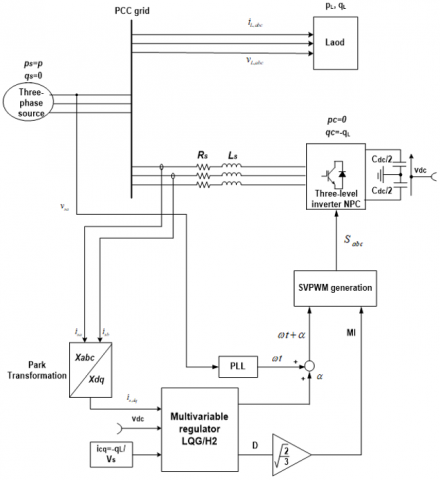Figure 1. Multi-variable control circuit of the STATCOM (corrected)

3. Control of the STATCOM

3.1 SVPWM strategy

Among the various modulation techniques for controlling the switches of a multi-level inverter is Space Vector Pulse Width Modulation (SVPWM). The latter directly employs the variable command provided by the control system and identifies each vector in the complex space (α, β) [18, 19]. Figure 2 shows the vector diagram in space for a three-level inverter.

There are six sectors (S1-S6), with four triangles(Δ0-Δ3) in each one, giving total of 33 which means 27 possible switching states for the three-level inverter.

All sectors of the three-level SVM outer hexagon can be split to four (4) small triangles, ordered as shown in Figure 2. Each voltage vector could be split into four groups, zero voltage vectors (000, 111, -1-1-1), small voltage vectors (110.00-1,…, 0-1-1), medium voltage vectors (10-1.01-1…, 1-10) and high voltage vectors (1-1-1.11-1,…, 1-11). All zero vectors are in the center of the hexagon, and the other voltage vectors (24 active voltage vectors) are on the corners of each triangle in the hexagon. All the possible states are shown in Figure 2 where a three-level inverter's vector diagram in space is divided into six (6) sectors. (Sector I, VI) Each sector is subdivided into four zones (Δ0, Δ1, Δ2, Δ3) .

## 2.pngFigure 2. Three-level inverter space vector diagram

According to the normalization, it is worth noting that the low voltage should be twice lower than that of the two-level SVM. In this manner, the same normalization is applied for the hexagon, hence, the voltages produce a reference vector and its position can be determined. Thus, the first normal sextant gives the results according to Figure 3.

## 3.png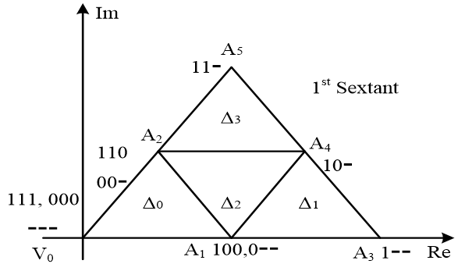Figure 3. The first normal sextant and the reference voltage vector

Each of these small triangles is then considered a two-level hexagon sector, with the same redundancy at the origin. The principle of the SVPWM is based upon the calculation of the control voltage vector by approximately using three adjacent vectors. In the case of sector I, the average reference voltage vector Vref for conventional two-level inverters is as follows [20, 21]:

$\left\{\begin{array}{c}T_{1} V_{1}+T_{2} V_{2}+T_{3} V_{3}=T_{S} V_{r e f} \\ T_{1}+T_{2}+T_{3}=T_{S}\end{array}\right.$    (6)

where V1, V2, and V3 are vectors which define the triangle region in which Vref is located. T1, T2 and T3 are the corresponding reference vector times and Ts is the switching period. The three-level inverter is similar to a two-level inverter, every space vector diagram is subdivided into 06 sectors. During this work, we will present the switching generation of switches for the sector I, so that the calculation method for other sectors is identical. Sector I is subdivided into four regions as shown in Figure 3, where all possible switching states for each region are also given. The SVPWM for three-level inverters can be implemented by employing the following steps: determining the sector, determining the region in the sector, calculating the switching times Ta, Tb and Tc, and finally determining the states of commutation [22, 23].

3.2 Synthesis of the LQG/H2 controller

Consider the Linear Time Invariant (LTI) system is illustrated by the state-space model as follows.

$\left\{\begin{array}{c}\dot{x}(t)=A x(t)+B u(t)+w(t) \\ y(t)=C x(t)+D(t) u(t)+v(t)\end{array}\right.$    (7)

where y(t) is the vector of outputs, A is the state matrix, B is the control or input matrix, C is the measurement or output matrix, and D is the direct transmission matrix.

This representation concerns only proper systems (i.e. which do not contain a pure derivative terms), for strictly proper systems, the matrix D=0. Let G(s) be the system's transfer function.

$G(s)=\left(\begin{array}{ll}A & B \\ C & D\end{array}\right)=C\left(s I_{n}-A\right)^{-1} B+D$    (8)

The control problem is easily derived in the time domain. It is assumed that the function G(s) has the following representation in state space:

$\left\{\begin{array}{c}\dot{x}(t)=A x(t)+B_{1} w(t)+B_{2} u(t) \\ z(t)=C_{1} x(t)+D_{11} w(t)+D_{12} u(t) \\ y(t)=C_{2} x(t)+D_{21} w(t)+D_{22} u(t)\end{array}\right.$    (9)

where $x \in R^{n}$  is the state vector, $w \in R^{n w}$  is disturbance acting on the input, $z \in R^{n z}$  is the controlled output, $u \in R^{n u}$  is the control signal, $y \in R^{n y}$  is the measured output. The matrices $A, B_{1}, B_{2}, C_{1}, C_{2}, D_{11}, D_{12}, D_{21}, D_{22}$  are constant matrices of appropriate size. It is assumed that the triplet ($A, B_{2}, C_{2}$) is stabilizable and detectable and $D_{11}=0$  and $D_{22}=0$.

The noises v(t) and w(t) on the state and on the output respectively are assumed to be Gaussian white noises of zero means and of variances equal respectively to V and W.

Consider the following quadratic criterion with Q and R symmetric positive definite matrices termed weighting matrices.

$J_{L Q G}=\lim _{t \rightarrow \infty} \frac{1}{T} \int_{0}^{\infty} E\left[x(t)^{T} Q x(t)+u(t)^{T} R u(t)\right] d t$

$=\lim _{t \rightarrow \infty} \int_{0}^{\infty} E\left[y_{1}(t)^{T} y_{1}(t)\right] d t$    (10)

$y=\left[\begin{array}{l}y_{11} \\ y_{12}\end{array}\right]=\left[\begin{array}{cc}Q^{1 / 2} & 0 \\ 0 & R^{1 / 2}\end{array}\right]\left[\begin{array}{l}x \\ u\end{array}\right]$    (11)

$u_{1}=\left[\begin{array}{l}u_{11} \\ u_{12}\end{array}\right]=\left[\begin{array}{cc}V^{1 / 2} & 0 \\ 0 & W^{1 / 2}\end{array}\right]^{-1}\left[\begin{array}{l}v \\ w\end{array}\right]$    (12)

Thus, the synthesis of a LQG regulator is only a particular case of the synthesis H2, for which one seeks a controller K(s) stabilizing the given augmented system depicted in Figure 4.

## 4.png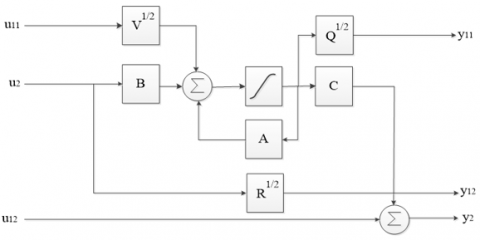Figure 4. Augmented system structure

The structure of Figure 2 is valid for strictly proper systems with D=0.

One solution to this problem is to measure the disturbances and the differences between the model and the process. This is the very principle of looping or feedback control, a block diagram of which is given in Figure 5 below.

## 5.png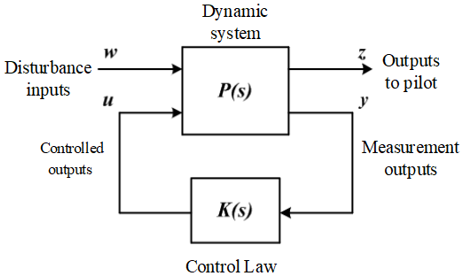Figure 5. Standard H2 problem

The matrix P in the state space being as follows:

$P(s)=\left[\begin{array}{ccc}A & B_{1} & B_{2} \\ C_{1} & 0 & D_{12} \\ C_{2} & D_{21} & 0\end{array}\right]=\left[\begin{array}{cccc}A & V^{1 / 2} & 0 & B \\ Q^{1 / 2} & 0 & 0 & 0 \\ 0 & 0 & 0 & V^{1 / 2} \\ 0 & 0 & W^{1 / 2} & 0\end{array}\right]$    (13)

and

$y_{1}=F_{1}(P, K) u_{1}$    (14)

The H2 norm of a w_2→z_2 transfer is an accurate measure of the output signal strength for a unitary white noise input. This is a criterion that measures the performance of disturbance power attenuation.

The system is represented as:

$\left[\begin{array}{l}z \\ y\end{array}\right]=\left[\begin{array}{ll}G_{11} & G_{12} \\ G_{21} & G_{22}\end{array}\right]\left[\begin{array}{l}v \\ u\end{array}\right]$    (15)

We call P the system (process) and the clean assumed. When this system is in closed loop with the control law u(t)=K(s).y(t), the transfer matrix between w and z of the loop system, can be easily obtained and is called Linear Fractional Transformation (LFT)) lower :

$F(P, K)=P_{11}+P_{12} K\left(I-K P_{22}\right)^{-1}$    (16)

The disturbances are assessed by using the signals from the measurement channel (vectors of the measurement outputs). This measurement makes it possible to attenuate the effect of disturbances on the output via the action channel formed by the control vector. The set point trajectory is then considered as a particular disturbance. The feedback makes it possible to reduce, within a certain limit, the differences between the process and the model .

LQG synthesis can be expressed in the frequency domain as the synthesis of a linear regulator invariant K stabilizer increased P system and minimizing the following criterion:

$J_{L Q G}=\left\|F_{1}(P, K)\right\|_{2}^{2}$    (17)

LQG control is designed within a stochastic context, i.e. when the matrices V and W correspond to variances. The resolution of H2 optimization problem requires the solution of the two following Riccati equations :

$X=\operatorname{Ric}\left[\begin{array}{cc}A-B_{2} D_{12}^{T} C_{1} & -B_{2} B_{2}^{T} \\ -M^{T} M & -\left(A-B_{2} D_{12}^{T} C_{1}\right)^{T}\end{array}\right]$    (18)

$Y=\operatorname{Ric}\left[\begin{array}{cc}-\left(A-B_{1} D_{21}^{T} C_{2}\right)^{T} & -C_{2} C_{2}^{T} \\ -N^{T} N & -A+B_{1} D_{21}^{T} C_{2}\end{array}\right]$    (19)

where X and Y are the stabilizing solutions to the ARE (Algebraic Riccati Equation).

With:

$M=\left(I-D_{12} D_{12}^{T}\right) C_{1}$    (20)

$N=B_{1}\left(I-D_{21}^{T} D_{21}\right)$    (21)

The optimal controller as defined in $J_{L Q G}$ criterion is then broken down into a structure observer / controller structure whose state feedback matrices F and observation H are given by:

$F=-\left(D_{12}^{T} C_{1}+B_{2}^{T} X\right)$    (22)

$H=-\left(B_{1} D_{21}^{T}+Y C_{2}^{T}\right)$    (23)

The use of a particular Y ou the parameterization of taking to the matrix controller K of the optimum state observer-controller structure defined by the matrices F and H are then obtained by the following structural matrix:

$K=\left[\begin{array}{ccc}A+B_{2} F+H C_{2} & -H & B_{2} \\ F & 0 & I \\ C_{2} & I & 0\end{array}\right]$    (24)

4. Simulation Results

In Table 1 of Appendix B, all of the simulation's components' parameters are mentioned. The overall model of the system and control scheme are implemented in MATLAB/Simulink. The circuit that is proposed is divided into two large sections: the first contains a three-phase rms voltage source of 380 V and a frequency of 50 Hz, a voltage source inverter controlled by space vector PWM in order to compensate for the reactive power generated by industrial loads. The second part is the control part which includes the multi-variable controller MIMO LQG/H2 and SVPWM technique.

In this simulation study two scenarios are presented and tested. The first scenario aims to compare the two and three level topologies of the STATCOM voltage source inverter and the second scenario aims to assess the control system's performance under changing references of reactive power.

4.1 Comparison between two and three level inverters

## 6.png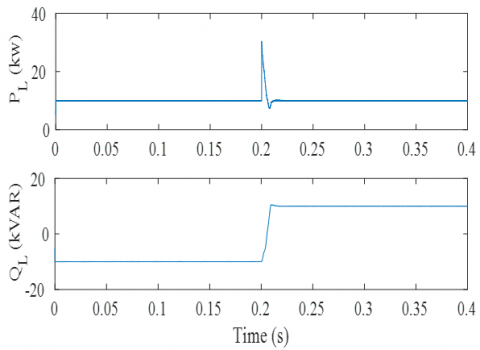Figure 6. The load’s active and reactive power

## 7a.png## 7b.pngFigure 7. The network's active and reactive power

## 8a.png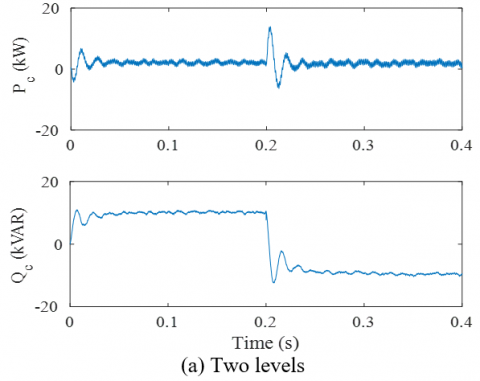## 8b.png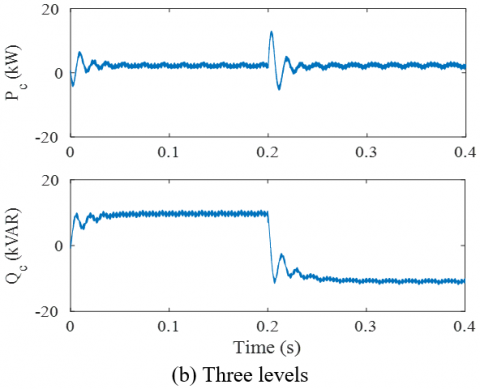Figure 8. The compensator’s active and reactive power

Figure 6 depicts the load's active and reactive powers. The active power of the load was fixed at 10 kW and the reactive power was varied from - 10 kVar to + 10 kVar.

The network's active and reactive power for the two-level and three-level inverters topologies are illustrated in Figure 7. The network provides the active power applied to the load. The reactive power becomes zero after a short transient. In addition, a significant improvement can be noticed on the time of the inverter answers to three levels at the two-level topology.

Figure 8 shows the instantaneous responses of the active and reactive powers of the compensator for two (Figure 8 (a)) and three levels (Figure 8 (b)). It can be seen that the active power becomes zero in steady state and the reactive power is inversely proportional to that of the load.

Figure 9 shows the waveforms of the network current $i_{s a}$, the load current $i_{L a}$  and that of the compensator $i_{c a}$, respectively with respect to the network voltage $v_{s a}$ for the two modes of inductive and capacitive load. It can be seen that the network current becomes in phase with its voltage.

The DC voltage $V_{d c}$, the modulation index MI and the phase difference between current and voltage of α network for the inverter to two level (a) and three levels (b) are shown in Figure 10. Voltage side is a constant ongoing follow its reference 800 V before and after the disturbance.

## 9a.png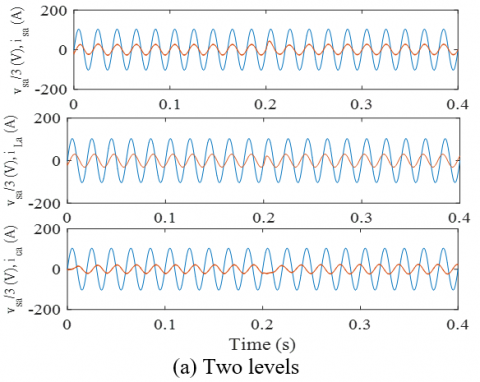## 9b.png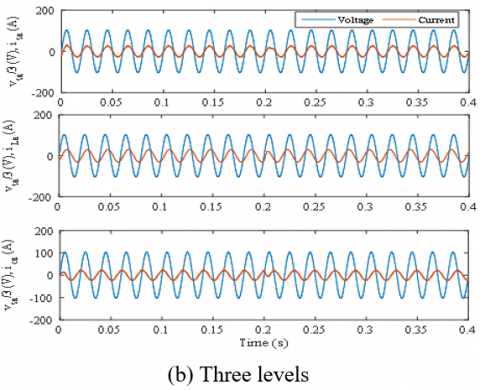Figure 9. The grid voltage $v_{s a}$, respectively with $i_{s a}$, $i_{L a}$ and $i_{c a}$

## 10a.png## 10b.pngFigure 10. The DC voltage and control laws

Figure 11 shows the Fourier series decomposition of the current of phase A of the network. A remarkable improvement in the shape of the current, which is well visualized at THD (3.63% for the three-level inverter compared to 4.24% for the two-level).

Scenario 02 consists of the variation of the load from 30 ÷ 10 kVAR and a permanent active power of 20 kW as shown by the Figure 12.

## 11a.png## 11b.png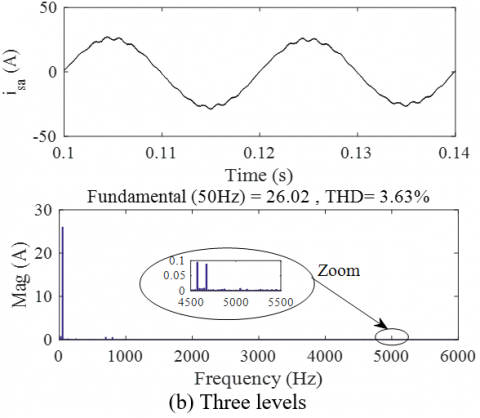Figure 11. The current of the phase A and its spectral analysis

## 12.png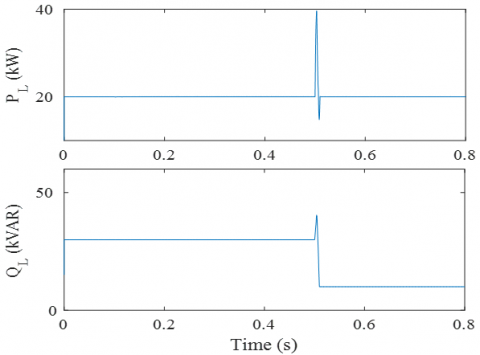Figure 12. The load’s active and reactive power

## 13.png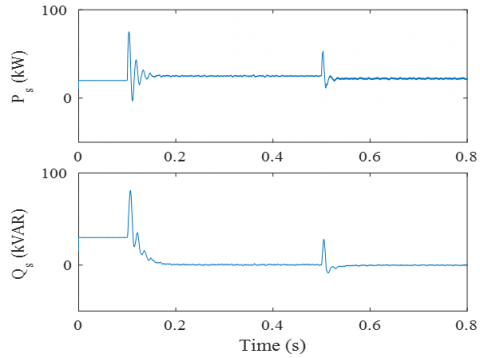Figure 13. The network’s active and reactive power

## 14.pngFigure 14. The compensator’s active and reactive power

The active and reactive power supplied by the network are shown in Figure 13. We notice that the reactive power of the network is canceled when the compensator starts up and that it goes through a very short transient regime after the sudden change in load.

Figure 14 shows the active power and reactive compensator. The active power is almost zero, as we can see. The reactive power is zero before starting the STATCOM. Once STATCOM is used, the compensator power is in the sign position relative to the load power to compensate for the reactive power requested by the load.

## 15.png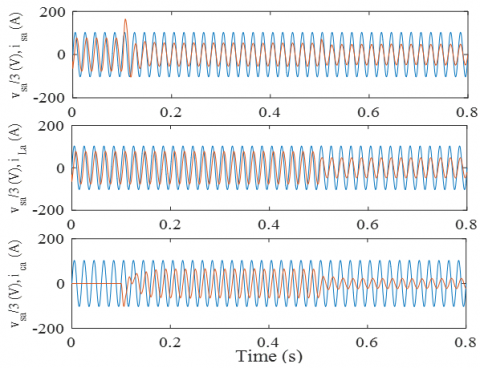Figure 15. The mains voltage $v_{s a}$, respectively with $i_{s a}$, $i_{L a}$ and $i_{c a}$

## 16.png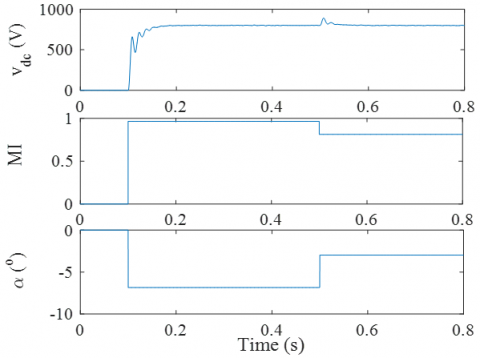Figure 16. The DC voltage and control laws

Figure 15 represents the waveforms of the current isa of the source, the current iLa of the load and that of the compensator ica, respectively with respect to the network voltage vsa for an inductive load mode. When the compensator is started at t = 0.1 s, we see that the network current becomes in phase with its voltage. At the instant t = 0.5 s, we applied a load of 10KVAR, noticing that despite this change the current isa remains in phase with its voltage vsa. It is thus noted that the current of the compensator ica is in phase opposition with the current of the load iLa for the purpose of compensate the reactive power.

Figure 16 shows the shape of the DC voltage and the control laws. The DC voltage is zero before starting the STATCOM. After using the compensator, the voltage continues to follow its reference despite the change in load. Modulation index changes are made to ensure DC voltage adjustment. The phase shift ensures the flow of reactive power with the aim of canceling it from the network.

Chen et al.  proposed a new passivity-based adaptive control (PBC: Passivity-Based Control) of a D-STATCOM-based cascade multilevel converter integrated with a medium voltage distribution system for power reactive compensation. Passivity based nonlinear control is designed to achieve the tracking of reference currents. In addition, adaptive control is also presented to mitigate the effects of disturbance and increase the performance of D-STATCOM. Experimental results are exposed and compared by Chen et al.  with those obtained by simulations in MATLAB/SIMULINK. The total harmonic distortion (THD) of the supply current is 3.46% for a seven-level inverter. However, several number of switches and DC voltage sources can increase the cost of STATCOM thus complicating switch control. To demonstrate the superiority and performance of the proposed control in this article with respect to the proposed advanced control method in Chen et al. . A new type of multilevel converter topology TIM (Triple Modular Inverter) has been proposed . Several benefits have been considered this structure except that it requires using a number of switches higher (18 switches). But for our topology uses a reduced number of switches (12 switches). The results obtained in this article (the THDs for the inverters with two and three levels are respectively 4.24 and 3.63%) are very satisfactory and the method used showed better performances in closed loop.

5. Conclusion

The presented study is a part in the research area of reactive energy compensation and energy quality, where we proposed an adequate solution to optimize the reactive power in an electrical network through the static power compensator STATCOM. Our work was devoted to the basic structure and the operating principle of STATCOM, as well as the multi-variable mathematical model in the synchronous frame (d-q) for the studied compensator when it is connected to the electrical network. We were oriented towards the Gaussian linear quadratic control LQG / H2. This command is based on a system state estimator with the use of the Kalman filter which plays two roles, one to identify system states and the other to filter out noisy measurements. The inverter switches were controlled by SVPWM technique. We managed to achieve a multi-variable control that shows better speed with the possibility to control the reactive component of the alternating current side and the DC side. Results of simulations performed using the MATLAB / Simulink software for STATCOM validated the general model of the used device. Finally, it was shown that the compensator was realized with high efficiency, which was our crucial goal.

Appendix

Appendix A

We have provided below a detailed derivation of the state-space model given by Eq. (5).

The current $I_{d c}$  through the DC link capacitor can be expressed in terms of the switching function S as:

$I_{d c}=S^{T} . I_{c a b c}$    (A.1)

where $I_{c a b c}$ is the three phase current of the compensator.

The representation of this current in the Park reference frame gives:

The compensator current in (dq) axes:

$\frac{d}{d t}\left[\begin{array}{l}I_{c d} \\ I_{c q}\end{array}\right]=\left[\begin{array}{cc}-\frac{R_{s}}{L_{s}} & \omega \\ -\omega & -\frac{R_{s}}{L_{s}}\end{array}\right]\left[\begin{array}{l}I_{c d} \\ I_{c q}\end{array}\right]+\frac{1}{L_{s}}\left[\begin{array}{c}V_{s} \cos (\alpha)-D V_{d c} \\ -V_{s} \sin (\alpha)\end{array}\right]$    (A.2)

The relationship between current and voltage on the DC side is written:

$I_{d c}=C_{s} . \frac{V_{d c}}{d t}$    (A.3)

$\frac{d V_{d c}}{d t}=\frac{D}{C_{s}} . I_{c d}$    (A.4)

The conversion coefficient in the Park D axes can be expressed by the following equation:

$D=\frac{\sqrt{V_{c d}^{2}+V_{c q}^{2}}}{V_{d c}}$    (A.5)

where vcd and vcq are the converter voltages.

And the phase angle α is given by:

$\alpha=\operatorname{arctg}\left(\frac{V_{o q}}{V_{o d}}\right)$    (A.6)

$\left\{\begin{array}{c}x(t+1)=A x(t)+B u(t) \\ y=C x(t)\end{array}\right.$    (A.7)

We obtain the complete mathematical model as follows .

With:

$A=\left[\begin{array}{ccc}-\frac{R_{s}}{L_{s}} & -\omega & 0 \\ 0 & -\frac{R_{s}}{L_{s}} & -\frac{D}{L_{s}} \\ 0 & \frac{D}{C_{s}} & 0\end{array}\right] ; B=\frac{1}{L_{s}}\left[\begin{array}{cc}-V_{s} & 0 \\ 0 & V_{d c 0} \\ 0 & 0\end{array}\right]$;

$C=\left[\begin{array}{ccc}-1 & 0 & 0 \\ 0 & 0 & 1\end{array}\right]$

$x=\left[\begin{array}{lll}i_{c q} & i_{c d} & i_{d c}\end{array}\right]^{T} ; u=\left[\begin{array}{ll}\alpha & D\end{array}\right]^{T}$

Appendix B

Table 1. Parameters of the Simulation Models

 Parameter Value Source voltage and frequency 380 V, 50 Hz Leakage impedence Rf, Lf 0.5 Ω, 1 mH SVPWM switching frequency, fs 2500 Hz Capacitance, Cs 1000 F Capacitor initial voltage, vdc0 800 V

The matrices of LQG/H2 regulator:

$A_{C}=10^{4}\left[\begin{array}{ccccc}0 & 0 & 0.0001 & 0 & 0 \\ 0 & 0 & 0 & 0 & -0.0001 \\ -3.9223 & 0.0252 & -3.9231 & -0.0265 & -0.0252 \\ 0.0531 & 8.2575 & 0.0532 & -8.3555 & -8.2668 \\ 0 & 0 & 0 & 0.0980 & 0\end{array}\right]$

$B_{C}=\left[\begin{array}{cc}0 & 0 \\ 0 & 0 \\ 0.0007 & 0 \\ -0.0001 & 0.0003 \\ 0 & -0.0021\end{array}\right]$

$C_{C}=\left[\begin{array}{ccccc}-0.8257 & 0.0053 & -0.8154 & 0.0010 & -0.0053 \\ -0.0053 & -0.8257 & -0.0022 & 0.8305 & 0.8218\end{array}\right]$

$D_{C}=\left[\begin{array}{ll}0 & 0 \\ 0 & 0\end{array}\right]$

References

 Jamhoria, S., Srivastava, L. (2014). Applications of thyristor controlled series compensator in power system: An overview. Conference of Power Signals Control and Computations (EPSCICON), pp. 1-6. https://doi.org/10.1109/EPSCICON.2014.6887516

 Šapurov, M., Bleizgys, V., Baskys, A., Dervinis, A., Bielskis, E., Paulikas, S., Macaitis, V. (2020). Asymmetric compensation of reactive power using thyristor-controlled reactors. Symmetry, 12(6): 880. https://doi.org/10.3390/sym12060880

 Gayatri, M.T.L., Parimi, A.M., Pavan Kumar, A.V. (2018). A review of reactive power compensation techniques in microgrids. Renewable and Sustainable Energy Reviews, 81: 1030-1036. https://doi.org/10.1016/j.rser.2017.08.006

 Panfilov, D.I., Rozhkov, A.N., Astashev, M.G., Zhuravlev, I.I. (2019). Modern approaches to controlled static VAR compensators design. In 2019 IEEE International Conference on Environment and Electrical Engineering and 2019 IEEE Industrial and Commercial Power Systems Europe (EEEIC/I&CPS Europe), pp. 1-5. https://doi.org/10.1109/EEEIC.2019.8783619

 Rasoulzadeh-Akhijahani, A., Mosallanejad, A. (2016). Analyzing TCSC and SVC eﬀects in wind power curtailment mitigation. Int. Trans. Electr. Energ. Syst., 26: 2445-2462. https://doi.org/10.1002/etep.2215

 Esparza, A., Segundo, J., Nunez, C., Visairo, N., Barocio, E., Garcia, H. (2018). Transient stability enhancement using a wide-area controlled SVC: An HIL validation approach. Energies, 11(7): 1639. https://doi.org/10.3390/en11071639.

 Kose, A., Irmak, E. (2016). Modeling and simulation of a static VAR compensator based on FC-TCR. 2016 IEEE International Conference on Renewable Energy Research and Applications (ICRERA). https://doi.org/10.1109/icrera.2016.7884470

 Kotsampopoulos, P., Georgilakis, P., Lagos, D.T., Kleftakis, V., Hatziargyriou, N. (2019). FACTS providing grid services: Applications and testing. Energies, 12(13): 2554. https://doi.org/10.3390/en1213255

 Farkoush, S., Kim, C.H., Rhee, S.B. (2016). THD Reduction of Distribution System Based on ASRFC and HVC Method for SVC under EV Charger Condition for Power Factor Improvement. Symmetry, 8(12): 156. https://doi.org/10.3390/sym8120156

 Mohamed, S.A., Luo, N., Pujol, T., Pacheco, L. (2018). Improvement of the performance stability of power grid with windfarms using static synchronous compensator. International Conference on Renewable Energies and Power Quality (ICREPQ’18) Salamanca (Spain). https://doi.org/10.24084/repqj16.212

 Yuvaraj, V., Deepa, S.N., Rozario, A.P.R., Kumar, M. (2011). Improving grid power quality with FACTS device on integration of wind energy system. 2011 Fifth Asia Modelling Symposium, pp. 157-162. https://doi.org/10.1109/ams.2011.38

 Liu, L., Wang, S.T. (2019). Improving low voltage ride-through with STATCOM and SDBR for wind turbine with squirrel-cage induction generator. European Journal of Electrical Engineering, 21(2): 179-187. https://doi.org/10.18280/ejee.210208

 Chavhan, S.T., Bhattar, C.L., Koli, P.V., Rathod, V.S. (2015). Application of STATCOM for power quality improvement of grid integrated wind mill. 2015 IEEE 9th International Conference on Intelligent Systems and Control (ISCO), pp. 1-7. https://doi.org/10.1109/isco.2015.7282295

 Ilango, K., Bhargav, A., Trivikram, A., Kavya, P.S., Mounika, G., Nair, M.G. (2012). Power quality improvement using STATCOM with renewable energy sources. 2012 IEEE 5th India International Conference on Power Electronics (IICPE), pp. 1-6. https://doi.org/10.1109/iicpe.2012.6450462

 Sundaram, A., Ashenafi P.F., Dawit A. (2016). Power quality improvement of electric grid connected wind-solar hybrid energy system using STATCOM. International Journal of Scientific Research in Science. 2(3): 905-909. ISSN: 2394-4099

 Ahsan, S., Siddiqui, A.S. (2016). Dynamic compensation of real and reactive power in wind farms using STATCOM. Perspectives in Science, 8: 519-521. https://doi.org/10.1016/j.pisc.2016.06.008

 Zhang T.Y., Li Y.D. (2019). A simplified pulse width modulation algorithm for model prediction of cascade static synchronous compensator. European Journal of Electrical Engineering, 21(1): 107-113. https://doi.org/10.18280/ejee.210116

 Merabet Boulouiha, H., Allali, A., Laouer, M., Tahri, A., Denaï, M., Draou, A. (2015). Direct torque control of multilevel SVPWM inverter in variable speed SCIG-based wind energy conversion system. Renewable Energy, 80: 140-152. https://doi.org/10.1016/j.renene.2015.01.065

 Fouad, K., Boulouiha, H. M., Allali, A., Taibi, A., Denai, M. (2016). Multivariable control of a grid-connected wind energy conversion system with power quality enhancement. Energy Systems, 9(1): 25-57. https://doi.org/10.1007/s12667-016-0223-7

 Boulouiha, H.M., Allali, A., Denai, M. (2017). Grid integration of wind energy systems: control design, stability, and power quality issues. In Clean Energy for Sustainable Development, pp. 239-335.

 Nair, M.D., Biswas, J., Vivek, G., Barai, M. (2019). Optimum hybrid SVPWM technique for three-level inverter on the basis of minimum RMS flux ripple. Journal of Power Electronics, 19(2): 413-430. https://doi.org/10.6113/JPE.2019.19.2.413

 Biswas, J., Nair, M. D., Gopinath, V., Barai, M. (2017). An optimized hybrid SVPWM strategy based on multiple division of active vector time (MDAVT). IEEE Transactions on Power Electronics, 32(6): 4607-4618. https://doi.org/10.1109/tpel.2016.2597247

 Ahmed, I., Borghate, V.B., Matsa, A., Meshram, P.M., Suryawanshi, H.M., Chaudhari, M.A. (2016). Simplified space vector modulation techniques for multilevel inverters. IEEE Transactions on Power Electronics, 31(12): 8483-8499. https://doi.org/10.1109/tpel.2016.2520078

 Sabatier, J., Lanusse, P., Melchior, P., Oustaloup, A. (2015). Fractional order differentiation and robust control design. Intelligent Systems, Control and Automation: Science and Engineering, 77: 13-18. https://doi.org/10.1007/978-94-017-9807-5

 Ishihara, T., Zheng, L.A. (2019). Reduced-order LQG/LTR procedure for the plant output side. Optimal Control Applications and Methods, 40(6): 1104-1119. https://doi.org/10.1002/oca.2539

 Chen, Y., Wen, M., Lei, E., Yin, X., Lai, J., Wang, Z. (2018). Passivity-based control of cascaded multilevel converter based D-STATCOM integrated with distribution transformer. Electric Power Systems Research, 154: 1-12. https://doi.org/10.1016/j.epsr.2017.08.001

 Pires, V.F., Cordeiro, A., Foito, D., Silva, J.F. (2019). A STATCOM based on a three-phase, triple inverter modular topology for multilevel operation. IEEE Transactions on Power Delivery, 34(5): 1988-1997. https://doi.org/10.1109/tpwrd.2019.2923087